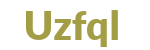# Artificial Intelligence Training

Artificial Intelligence TrainingTotal Learners

0 LearnersCourse Duration

0 Hours

## Artificial Intelligence Training Course Syllabus

• Learn Descriptive and Inferential statistics concepts and implementation using real-world data will also be covered. It will end with a case study implementation of EDA.

• Statistics Fundamentals and Implementation
• Exploratory Data Analysis
• Python is a critical tool for Data Science. In this module participants learn Python programming from basic to advanced level using Jupyter notebooks. Here, participants create, subset and manipulate various data structures. Specific libraries like NumPy, Pandas and Matplotlib that are popular for Data Analysis are covered in depth.

• ESSENTIALS OF PYTHON PROGRAMMING
• BASIC DATA STRUCTURES AND FUNCTIONS IN PYTHON
• INTRODUCTION TO
-Numpy Library
-Pandas Library
-Matplotlib and Seaborn Library
• DATA EXPLORATION USING STATISTICS
• Machine Learning algorithms are the backbone of Predictive Modelling. This is where the Crux of Data Science lies. The end objective of solving a data science problem is finding the patterns in the data and represent that in the form of a Data model. The algorithms taught in our course cover almost all of the problems data scientists solve on a regular basis.

• Introduction to Supervised and Unsupervised Learning
• Linear Regression with Multiple Variables
• Logistic Regression
• Decision Trees [CART]
• k-Fold Cross Validation
• Bagging and Bootstrapping
• Random Forest
• Principal component Analysis
• K-means clustering
• Hierarchical Clustering
• KNN
• Support Vector Machine
• Naive Bayes
• Time Series Analysis
• In this module, participants will learn the foundations of Deep Learning and understand how to build neural networks. The implementation of the same will be done using Python, TensorFlow and Keras.

• Introduction to Artificial Neural networks with Keras
• From Biological to Artificial Neurons
• Implementing MLPs with Keras
• Fine-Tuning Neural Network Hyperparameters
• Training Deep Neural Networks
• Reusing Pretrained Layers
• Faster Optimizers
• Avoiding Overfitting Through Regularization
• Summary and Practical Guidelines
• Take a step further in Deep Learning in this module with Convolutional Neural Networks. Learn how to implement Customize Models, Train Algorithms and implement Object Detection.

• Custom Models and Training with TensorFlow
• Deep Computer Vision Using Convolutional Neural Networks

## Artificial Intelligence Training Ratings

### 0

#### 0

H2K Infosys - IT Training & Placement Assistance
Rated 4.8/5 based on 198 customer reviews

### Request a DemoI agree to the H2KInfosys T&C